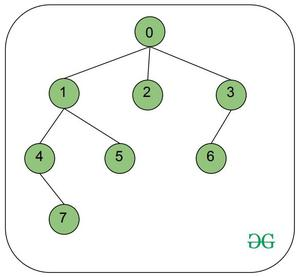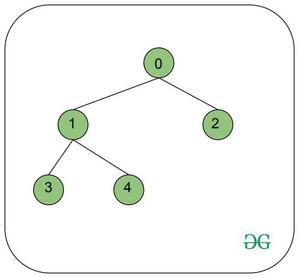# Sum of Nodes and respective Neighbors on the path from root to a vertex V

Given a rooted tree having N vertices, an array values[ ], which represents the value assigned to each node, and a vertex V, the task is to calculate the sum of values of nodes and immediate neighbors lying in the path from the root(always 0) to V.

Examples:

Input: N = 8, values = {1, 2, 3, 0, 0, 4, 3, 6}, V = 7Output: 16
Explanation:
Path from root (0) to V (7) = 0 -> 1 -> 4 -> 7
Neighbors of 0 = (2, 3), Sum = 1(node 0) + 3(node 2) + 0(node 3) = 4
Neighbors of 1 = (5), Sum = 2(node 1) + 4(node 5) = 6
No neighbor of 4, Sum = 0(node 4) = 0
No neighbor of 7, Sum = 6(node 7) = 6
Total sum = 4 + 6 + 0 + 6 = 16

Input: N = 5, values = {5, 6, 2, 9, 0}, V = 2Output: 13

## Recommended: Please try your approach on {IDE} first, before moving on to the solution.

Approach:

The idea is to store the parent of each node in an array and add the value of each parent with its child and store it in the parent node. Now, each node will hold sum of its value and value of corresponding neighbors. Use this array to find the required sum of the path from root to vertex V.

Follow the steps below to solve the problem:

• Initialize an array to store the values of each node and its corresponding neighbors using DFS Traversal.
• Iterate from vertex V to root using the array and keep adding the value of all the nodes found on the path.
• Finally, print the obtained sum.

Below is the implementation of the above approach:

## Java

 `// Java Program to implement ` `// the above appraoch ` `import` `java.io.*; ` `import` `java.util.*; ` ` `  `class` `GFG { ` ` `  `    ``// Creating Adjacency list ` `    ``private` `static` `List > ` `    ``constructTree(``int` `n, ``int``[][] edges) ` `    ``{ ` ` `  `        ``List > adjl ` `            ``= ``new` `ArrayList >(); ` ` `  `        ``for` `(``int` `i = ``0``; i < n; i++) { ` ` `  `            ``adjl.add(``new` `ArrayList()); ` `        ``} ` ` `  `        ``for` `(``int``[] e : edges) { ` `            ``int` `u = e[``0``]; ` `            ``int` `v = e[``1``]; ` `            ``adjl.get(u).add(v); ` `            ``adjl.get(v).add(u); ` `        ``} ` ` `  `        ``return` `adjl; ` `    ``} ` ` `  `    ``// Function to perform DFS traversal ` `    ``private` `static` `void` `DFS( ` `        ``List > adjl, ` `        ``int``[] parent, ``int` `u, ``int` `p) ` `    ``{ ` ` `  `        ``// Initializing parent of each node to p ` `        ``parent[u] = p; ` ` `  `        ``// Iterate over the children ` `        ``for` `(``int` `v : adjl.get(u)) { ` ` `  `            ``if` `(v != p) { ` ` `  `                ``DFS(adjl, parent, v, u); ` `            ``} ` `        ``} ` `    ``} ` ` `  `    ``// Function to add values of children to ` `    ``// respective parent nodes ` `    ``private` `static` `int``[] valuesFromChildren( ` `        ``int``[] parent, ``int``[] values) ` `    ``{ ` ` `  `        ``int``[] valuesChildren ` `            ``= ``new` `int``[parent.length]; ` ` `  `        ``for` `(``int` `i = ``0``; i < parent.length; i++) { ` ` `  `            ``// Root node ` `            ``if` `(parent[i] == -``1``) ` `                ``continue``; ` ` `  `            ``else` `{ ` `                ``int` `p = parent[i]; ` ` `  `                ``valuesChildren[p] += values[i]; ` `            ``} ` `        ``} ` `        ``return` `valuesChildren; ` `    ``} ` ` `  `    ``// Function to return sum of values of ` `    ``// each node in path from V to the root ` `    ``private` `static` `int` `findSumOfValues( ` `        ``int` `v, ``int``[] parent, ` `        ``int``[] valuesChildren) ` `    ``{ ` ` `  `        ``int` `cur_node = v; ` `        ``int` `sum = ``0``; ` ` `  `        ``// Path from node V to root node ` `        ``while` `(cur_node != -``1``) { ` ` `  `            ``sum += valuesChildren[cur_node]; ` `            ``cur_node = parent[cur_node]; ` `        ``} ` ` `  `        ``return` `sum; ` `    ``} ` ` `  `    ``// Driver Code ` `    ``public` `static` `void` `main(String[] args) ` `    ``{ ` ` `  `        ``int` `n = ``8``; ` ` `  `        ``// Insert edges into the graph ` `        ``int``[][] edges = { { ``0``, ``1` `}, ` `                          ``{ ``0``, ``2` `}, ` `                          ``{ ``0``, ``3` `}, ` `                          ``{ ``1``, ``4` `}, ` `                          ``{ ``1``, ``5` `}, ` `                          ``{ ``4``, ``7` `}, ` `                          ``{ ``3``, ``6` `} }; ` ` `  `        ``int` `v = ``7``; ` ` `  `        ``// Values assigned to each vertex ` `        ``int``[] values = ``new` `int``[] { ``1``, ``2``, ``3``, ``0``, ` `                                   ``0``, ``4``, ``3``, ``6` `}; ` ` `  `        ``// Constructing the tree ` `        ``// using adjacency list ` `        ``List > adjl ` `            ``= constructTree(n, edges); ` ` `  `        ``// Parent array ` `        ``int``[] parent = ``new` `int``[n]; ` ` `  `        ``// store values in the parent array ` `        ``DFS(adjl, parent, ``0``, -``1``); ` ` `  `        ``// Add values of children to the parent ` `        ``int``[] valuesChildren ` `            ``= valuesFromChildren(parent, values); ` ` `  `        ``// Find sum of nodes lying in the path ` `        ``int` `sum = findSumOfValues( ` `            ``v, parent, ` `            ``valuesChildren); ` ` `  `        ``// Add root node since ` `        ``// its value is not included yet ` `        ``sum += values[``0``]; ` ` `  `        ``System.out.println(sum); ` `    ``} ` `} `

Output:

```16
```

Time Complexity: O(N)
Auxiliary Space: O(N)

Attention reader! Don’t stop learning now. Get hold of all the important DSA concepts with the DSA Self Paced Course at a student-friendly price and become industry ready.

My Personal Notes arrow_drop_upCheck out this Author's contributed articles.

If you like GeeksforGeeks and would like to contribute, you can also write an article using contribute.geeksforgeeks.org or mail your article to contribute@geeksforgeeks.org. See your article appearing on the GeeksforGeeks main page and help other Geeks.

Please Improve this article if you find anything incorrect by clicking on the "Improve Article" button below.